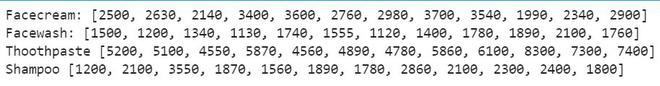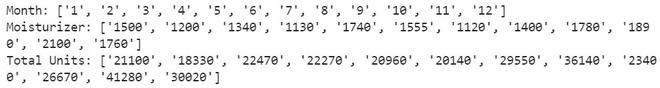# Python – Read CSV Columns Into List

• Difficulty Level : Medium
• Last Updated : 17 Sep, 2021

CSV file stores tabular data (numbers and text) in plain text. Each line of the file is a data record. Each record consists of one or more fields, separated by commas. The use of the comma as a field separator is the source of the name for this file format. In this article, we will read data from a CSV file into a list. We will use the panda’s library to read the data into a list.

File Used: file.

Attention geek! Strengthen your foundations with the Python Programming Foundation Course and learn the basics.

To begin with, your interview preparations Enhance your Data Structures concepts with the Python DS Course. And to begin with your Machine Learning Journey, join the Machine Learning - Basic Level Course

Method 1: Using Pandas

Here, we have the read_csv() function which helps to read the CSV file by simply creating its object. The column name can be written inside this object to access a particular column, the same as we do in accessing the elements of the array. Pandas library has a function named as tolist() that converts the data into a list that can be used as per our requirement. So, we will use this to convert the column data into a list. Finally, we will print the list.

Approach:

• Import the module.
• Read data from CSV file.
• Convert it into the list.
• Print the list.

Below is the implementation:

## Python3

 `# importing module` `from` `pandas ``import` `*`   `# reading CSV file` `data ``=` `read_csv(``"company_sales_data.csv"``)`   `# converting column data to list` `month ``=` `data[``'month_number'``].tolist()` `fc ``=` `data[``'facecream'``].tolist()` `fw ``=` `data[``'facewash'``].tolist()` `tp ``=` `data[``'toothpaste'``].tolist()` `sh ``=` `data[``'shampoo'``].tolist()`   `# printing list data` `print``(``'Facecream:'``, fc)` `print``(``'Facewash:'``, fw)` `print``(``'Toothpaste:'``, tp)` `print``(``'Shampoo:'``, sh)`

Output:Method 2: Using csv module

In this method we will import the csv library and open the file in reading mode, then we will use the DictReader() function to read the data of the CSV file. This function is like a regular reader, but it maps the information to a dictionary whose keys are given by the column names and all the values as keys. We will create empty lists so that we can store the values in it. Finally, we access the key values and append them into the empty lists and print that list.

## Python3

 `# importing the module` `import` `csv`   `# open the file in read mode` `filename ``=` `open``(``'company_sales_data.csv'``, ``'r'``)`   `# creating dictreader object` `file` `=` `csv.DictReader(filename)`   `# creating empty lists` `month ``=` `[]` `totalprofit ``=` `[]` `totalunit ``=` `[]`   `# iterating over each row and append` `# values to empty list` `for` `col ``in` `file``:` `    ``month.append(col[``'month_number'``])` `    ``totalprofit.append(col[``'moisturizer'``])` `    ``totalunit.append(col[``'total_units'``])`   `# printing lists` `print``(``'Month:'``, month)` `print``(``'Moisturizer:'``, totalprofit)` `print``(``'Total Units:'``, totalunit)`

Output:My Personal Notes arrow_drop_up
Recommended Articles
Page :